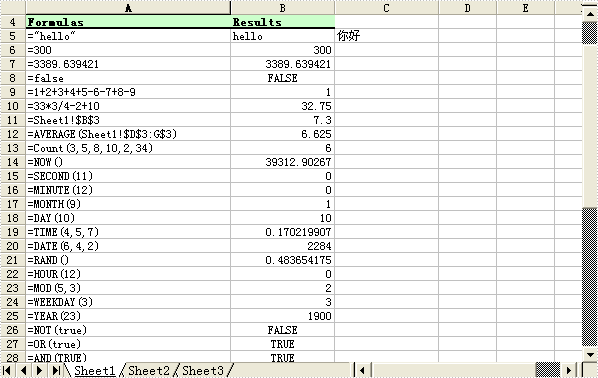Introduction

In an Excel Worksheet, we may import a great deal of data. Sometimes, we need to calculate the data to get another numbers we need. Formula is the mail tool to calculate data.

There are various formulas included in Excel. Actually, formulas are equations and each one starts with an equal sign. We can use a formula to calculate values for a column list. A formula contains four parts: functions, column references, operators and constants.

How to Add Formula for Excel in MS

There are different methods to add a formula for Excel including different part. This example is about formulas containing functions. The example is about how to average all number in the range from A1 to B4.

Firstly, click the cell where we wan to add the formula. Then, click Insert Function on the formula bar. Thirdly, select the function we want to use. We can search the function or browse form the categories. Next, enter the arguments. Click Collapse Dialog to hide the dialog box to enter cell references as an argument. Press Expand Dialog after selecting the cells on the worksheet. Finally, Press Enter when we complete the formula.

How to Add Formula for Excel with Spire.XLS

Spire.XLS presents you an easiest way to add formula for Excel. We will give you a demo with many kinds of formulas written in the worksheet. The formula can be string, bool value, calculation, sheet area reference, time and so on. Here, we reference a variable "currentRow" to control rows of all kinds of formulas. You may add formula text with the property of sheet.Range[++currentRow, 1].Text, and calculate the formula with the property of sheet.Range[currentRow, 2].Formula.

The following code shows how to add a formula for Excel with C#/VB.NET:

[C#]

using Spire.Xls;

namespace Formula

{

class Program

{

static void Main(string[] args)

{

//Create a new workbook

Workbook workbook = new Workbook();

//Initialize worksheet

Worksheet sheet = workbook.Worksheets;

//initialize currentRow

int currentRow = 3;

string currentFormula = string.Empty;

//test data

sheet.Range[currentRow, 2].NumberValue = 7.3;

sheet.Range[currentRow, 3].NumberValue = 5; ;

sheet.Range[currentRow, 4].NumberValue = 8.2;

sheet.Range[currentRow, 5].NumberValue = 4;

sheet.Range[currentRow, 6].NumberValue = 3;

sheet.Range[currentRow, 7].NumberValue = 11.3;

//string.

currentFormula = "=\"hello\"";

sheet.Range[++currentRow, 1].Text = "=\"hello\"";

sheet.Range[currentRow, 2].Formula = currentFormula;

//bool.

currentFormula = "=false";

sheet.Range[++currentRow, 1].Text = currentFormula;

sheet.Range[currentRow, 2].Formula = currentFormula;

//calculation

currentFormula = "=33*3/4-2+10";

sheet.Range[++currentRow, 1].Text = currentFormula;

sheet.Range[currentRow, 2].Formula = currentFormula;

//sheet area reference

currentFormula = "=AVERAGE(Sheet1!\$D\$3:G\$3)";

sheet.Range[++currentRow, 1].Text = currentFormula;

sheet.Range[currentRow, 2].Formula = currentFormula;

//time

currentFormula = "=NOW()";

sheet.Range[++currentRow, 1].Text = currentFormula;

sheet.Range[currentRow, 2].Formula = currentFormula;

sheet.Range[currentRow, 2].Style.NumberFormat = "yyyy-MM-DD";

//Save the file

workbook.SaveToFile("Sample.xls");

//Launch the file

System.Diagnostics.Process.Start("Sample.xls");

}

}

}

[Visual Basic]

Imports Spire.Xls

Module Module1

Sub Main()

'Create a new workbook

Dim workbook As New Workbook()

'Initialize worksheet

Dim sheet As Worksheet = workbook.Worksheets(0)

'initialize currentRow

Dim currentRow As Integer = 3

Dim currentFormula As String = String.Empty

'test data

sheet.Range(currentRow, 2).NumberValue = 7.3

sheet.Range(currentRow, 3).NumberValue = 5

sheet.Range(currentRow, 4).NumberValue = 8.2

sheet.Range(currentRow, 5).NumberValue = 4

sheet.Range(currentRow, 6).NumberValue = 3

sheet.Range(currentRow, 7).NumberValue = 11.3

'string.

currentFormula = "=""hello"""

sheet.Range(System.Threading.Interlocked.Increment(currentRow), 1).Text = "=""hello"""

sheet.Range(currentRow, 2).Formula = currentFormula

'bool.

currentFormula = "=false"

sheet.Range(System.Threading.Interlocked.Increment(currentRow), 1).Text = currentFormula

'calculation

currentFormula = "=33*3/4-2+10"

sheet.Range(System.Threading.Interlocked.Increment(currentRow), 1).Text = currentFormula

sheet.Range(currentRow, 2).Formula = currentFormula

'sheet area reference

currentFormula = "=AVERAGE(Sheet1!\$D\$3:G\$3)"

sheet.Range(System.Threading.Interlocked.Increment(currentRow), 1).Text = currentFormula

sheet.Range(currentRow, 2).Formula = currentFormula

'time

currentFormula = "=NOW()"

sheet.Range(System.Threading.Interlocked.Increment(currentRow), 1).Text = currentFormula

sheet.Range(currentRow, 2).Formula = currentFormula

sheet.Range(currentRow, 2).Style.NumberFormat = "yyyy-MM-DD"

'Save doc file.

workbook.SaveToFile("Sample.xls")

'Launching the MS Word file.

System.Diagnostics.Process.Start("Sample.xls")

End Sub

End Module

After running the demo, you will find the formula in the worksheet: